## 二叉查找树（Binary Search Tree）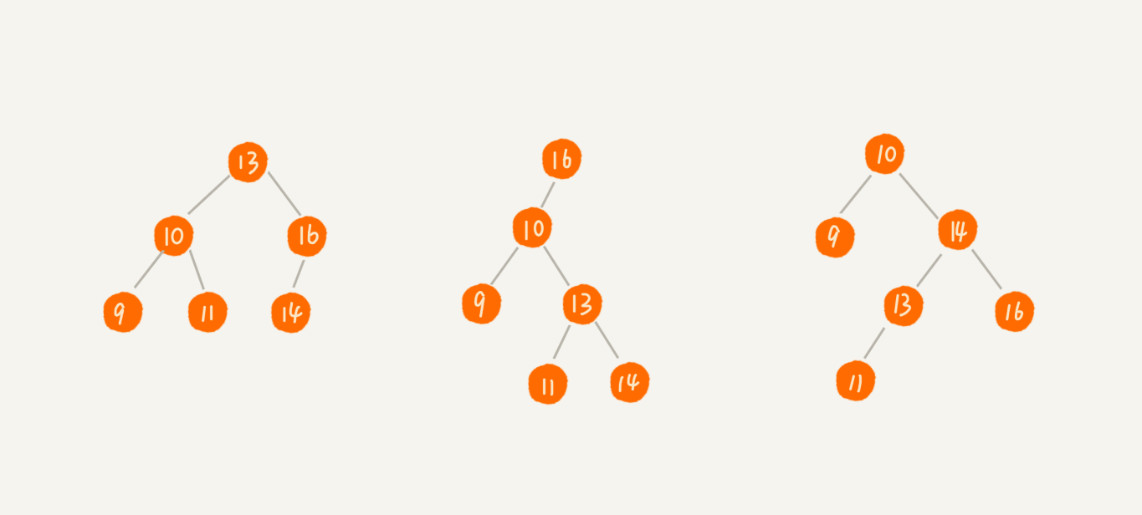1. 二叉查找树的查找操作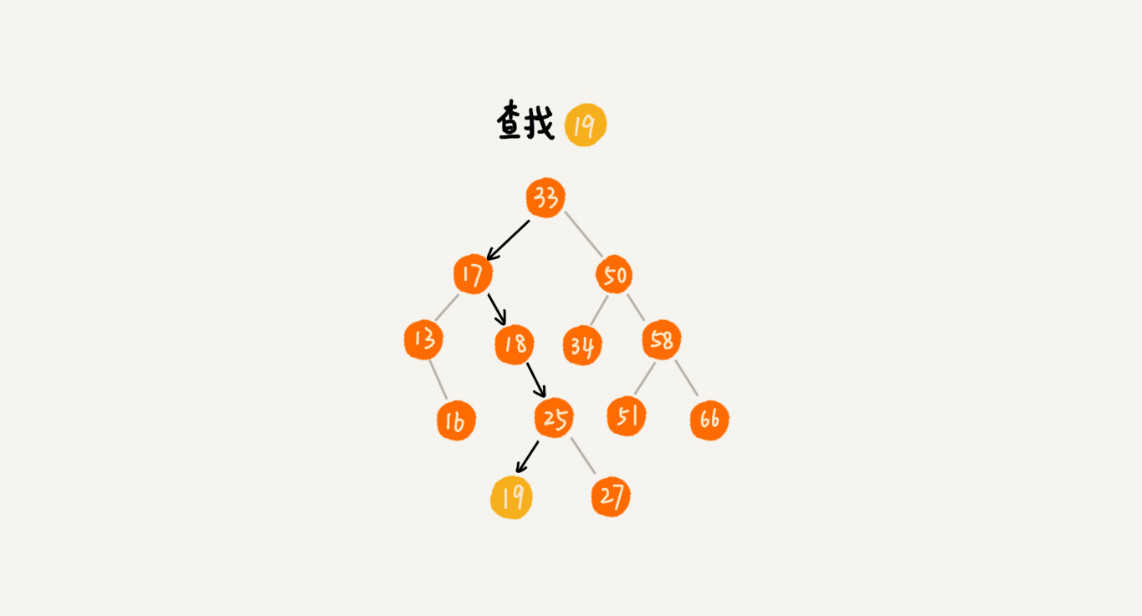``````public class BinarySearchTree {
private Node tree;

public Node find(int data) {
Node p = tree;
while (p != null) {
if (data < p.data) p = p.left;
else if (data > p.data) p = p.right;
else return p;
}
return null;
}

public static class Node {
private int data;
private Node left;
private Node right;

public Node(int data) {
this.data = data;
}
}
}``````

2. 二叉查找树的插入操作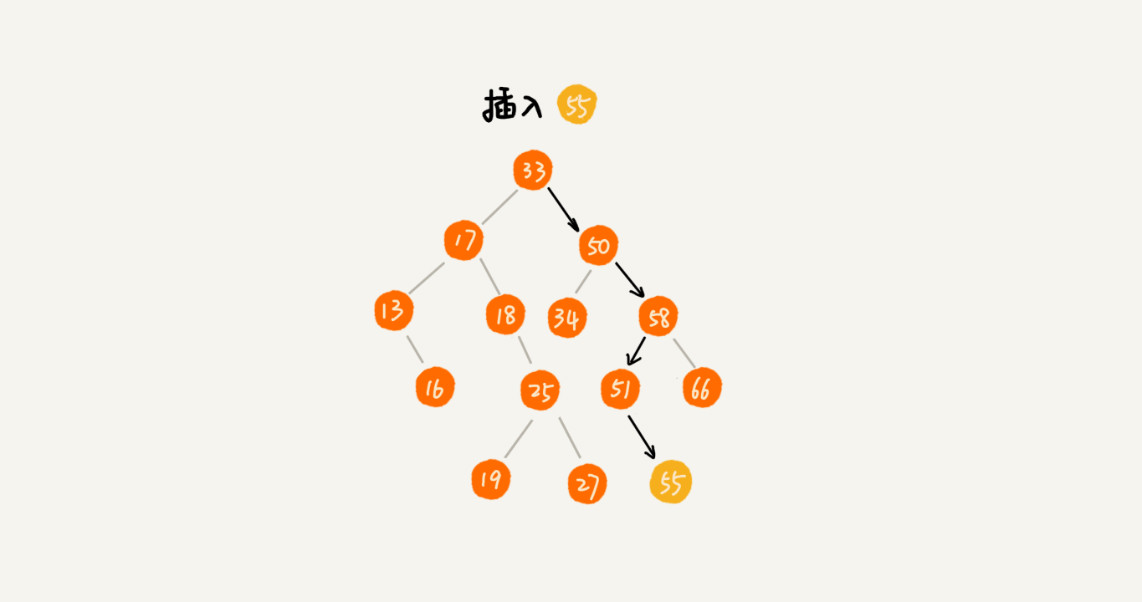``````public void insert(int data) {
if (tree == null) {
tree = new Node(data);
return;
}

Node p = tree;
while (p != null) {
if (data > p.data) {
if (p.right == null) {
p.right = new Node(data);
return;
}
p = p.right;
} else { // data < p.data
if (p.left == null) {
p.left = new Node(data);
return;
}
p = p.left;
}
}
}``````

3. 二叉查找树的删除操作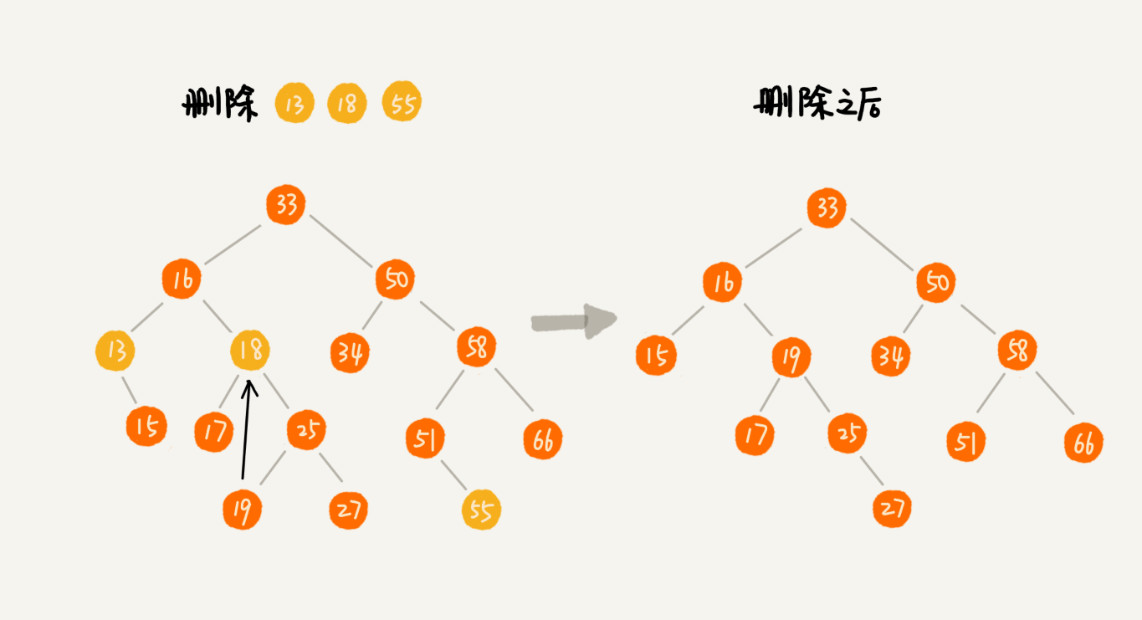``````public void delete(int data) {
Node p = tree; // p 指向要删除的节点，初始化指向根节点
Node pp = null; // pp 记录的是 p 的父节点
while (p != null && p.data != data) {
pp = p;
if (data > p.data) p = p.right;
else p = p.left;
}
if (p == null) return; // 没有找到

// 要删除的节点有两个子节点
if (p.left != null && p.right != null) { // 查找右子树中最小节点
Node minP = p.right;
Node minPP = p; // minPP 表示 minP 的父节点
while (minP.left != null) {
minPP = minP;
minP = minP.left;
}
p.data = minP.data; // 将 minP 的数据替换到 p 中
p = minP; // 下面就变成了删除 minP 了
pp = minPP;
}

// 删除节点是叶子节点或者仅有一个子节点
Node child; // p 的子节点
if (p.left != null) child = p.left;
else if (p.right != null) child = p.right;
else child = null;

if (pp == null) tree = child; // 删除的是根节点
else if (pp.left == p) pp.left = child;
else pp.right = child;
}``````

4. 二叉查找树的其他操作

## 支持重复数据的二叉查找树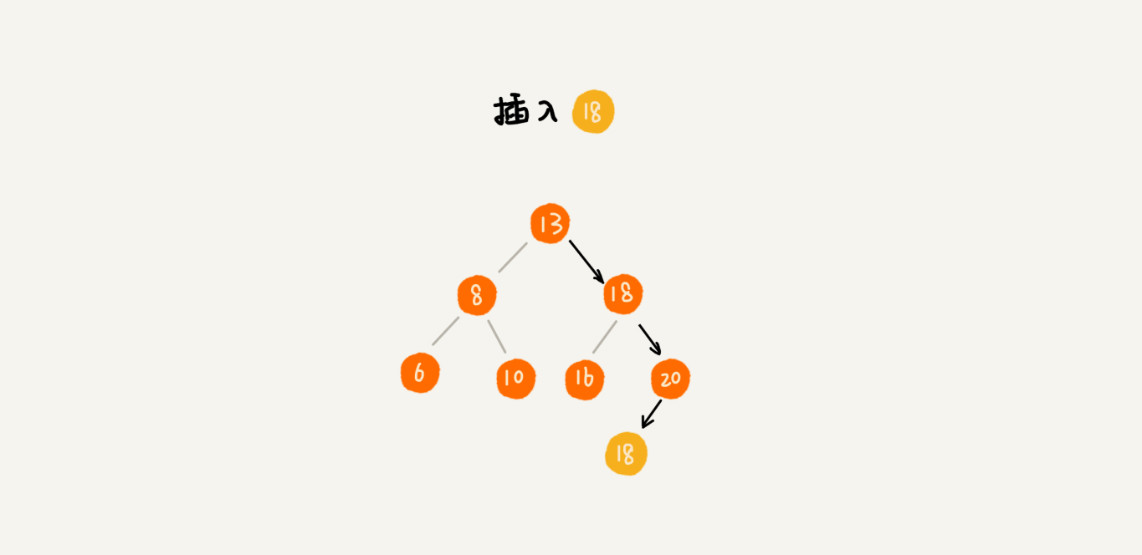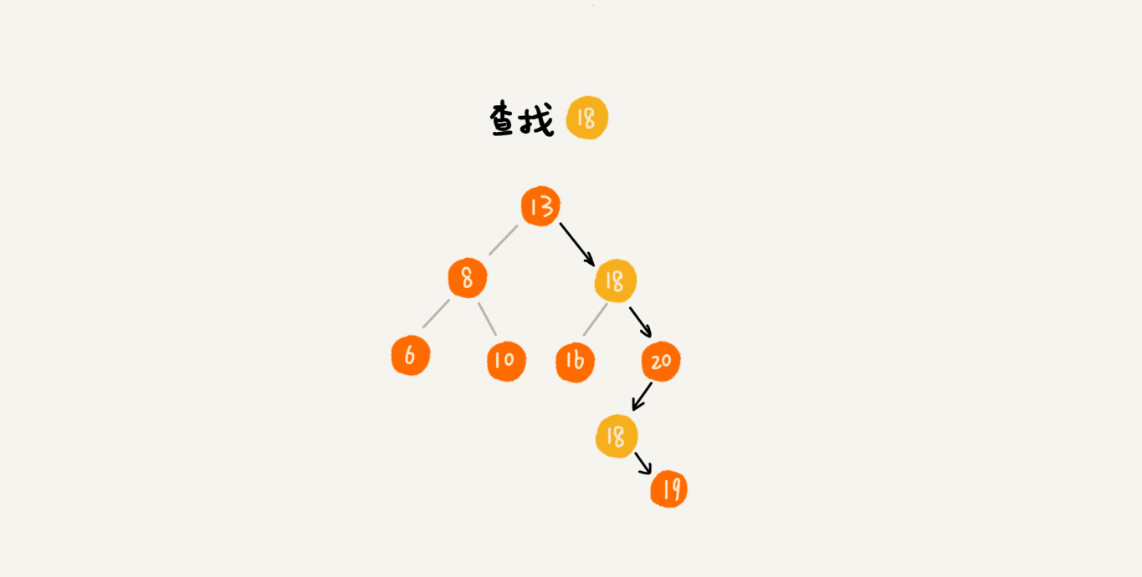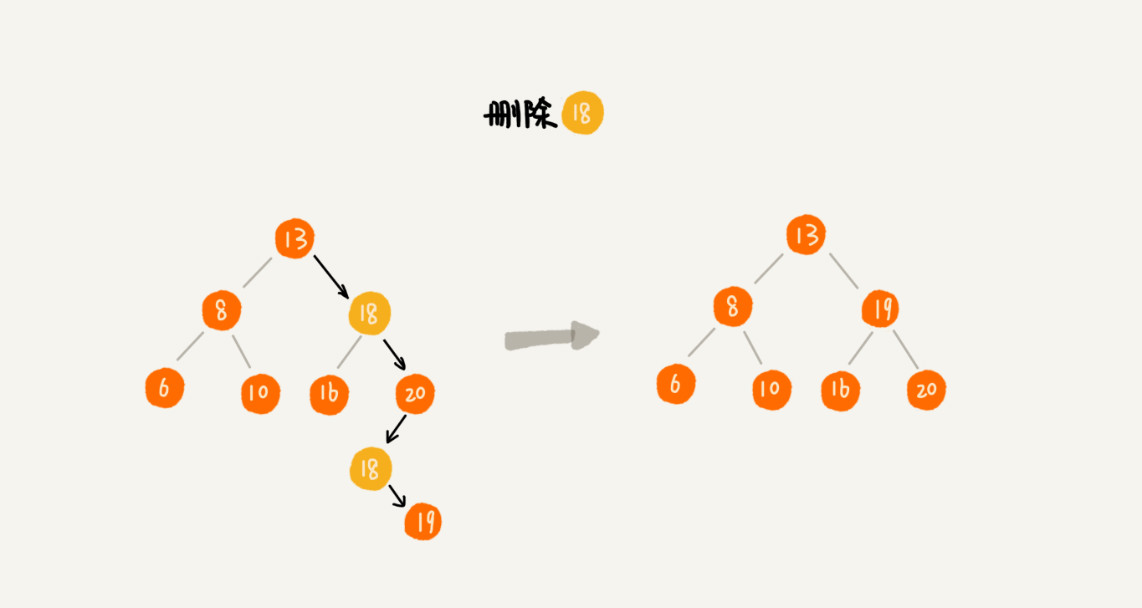## 二叉查找树的时间复杂度分析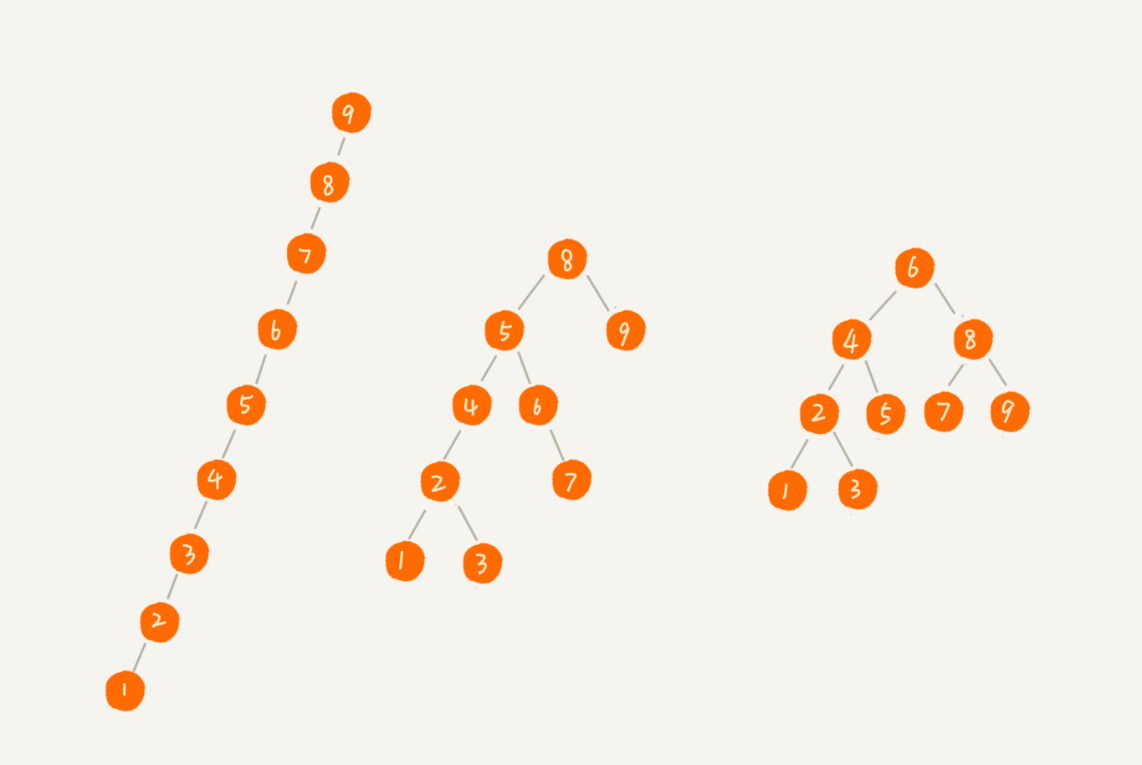``````n >= 1+2+4+8+...+2^(L-2)+1
n <= 1+2+4+8+...+2^(L-2)+2^(L-1)``````

## 思考

• 散列表的插入、删除、查找操作的时间复杂度可以做到常量级的 \$O(1)\$，非常高效。而二叉查找树在比较平衡的情况下，插入、删除、查找操作时间复杂度才是 \$O(logn)\$，相对散列表，好像并没有什么优势，那为什么还要用二叉查找树呢？

有下面几个原因：

第一，散列表中的数据是无序存储的，如果要输出有序的数据，需要先进行排序。而对于二叉查找树来说，只需要中序遍历，就可以在 \$O(n)\$ 的时间复杂度内，输出有序的数据序列。

第二，散列表扩容耗时很多，而且当遇到散列冲突时，性能不稳定，尽管二叉查找树的性能不稳定，但是在工程中，最常用的平衡二叉查找树的性能非常稳定，时间复杂度稳定在 \$O(logn)\$。

第三，笼统地来说，尽管散列表的查找等操作的时间复杂度是常量级的，但因为哈希冲突的存在，这个常量不一定比 `logn` 小，所以实际的查找速度可能不一定比 \$O(logn)\$ 快。加上哈希函数的耗时，也不一定就比平衡二叉查找树的效率高。

第四，散列表的构造比二叉查找树要复杂，需要考虑的东西很多。比如散列函数的设计、冲突解决办法、扩容、缩容等。平衡二叉查找树只需要考虑平衡性这一个问题，而且这个问题的解决方案比较成熟、固定。

最后，为了避免过多的散列冲突，散列表装载因子不能太大，特别是基于开放寻址法解决冲突的散列表，不然会浪费一定的存储空间。

综合这几点，平衡二叉查找树在某些方面还是优于散列表的，所以，这两者的存在并不冲突。在实际的开发过程中，需要结合具体的需求来选择使用哪一个。

• 如何通过编程，求出一棵给定二叉树的确切高度呢？

确定二叉树高度有两种思路：
第一种是深度优先思想的递归，分别求左右子树的高度。当前节点的高度就是左右子树中较大的那个`+1`
第二种可以采用层次遍历的方式，每一层记录都记录下当前队列的长度，这个是队尾，每一层队头从`0`开始。然后每遍历一个元素，队头下标`+1`。直到队头下标等于队尾下标。这个时候表示当前层遍历完成。每一层刚开始遍历的时候，树的高度`+1`。最后队列为空，就能得到树的高度。

## 二叉查找树的完整实现

``````public class BinarySearchTree {
private Node tree;

public Node find(int data) {
Node p = tree;
while (p != null) {
if (data < p.data) p = p.left;
else if (data > p.data) p = p.right;
else return p;
}
return null;
}

public void insert(int data) {
if (tree == null) {
tree = new Node(data);
return;
}

Node p = tree;
while (p != null) {
if (data > p.data) {
if (p.right == null) {
p.right = new Node(data);
return;
}
p = p.right;
} else { // data < p.data
if (p.left == null) {
p.left = new Node(data);
return;
}
p = p.left;
}
}
}

public void delete(int data) {
Node p = tree; // p指向要删除的节点，初始化指向根节点
Node pp = null; // pp记录的是p的父节点
while (p != null && p.data != data) {
pp = p;
if (data > p.data) p = p.right;
else p = p.left;
}
if (p == null) return; // 没有找到

// 要删除的节点有两个子节点
if (p.left != null && p.right != null) { // 查找右子树中最小节点
Node minP = p.right;
Node minPP = p; // minPP表示minP的父节点
while (minP.left != null) {
minPP = minP;
minP = minP.left;
}
p.data = minP.data; // 将minP的数据替换到p中
p = minP; // 下面就变成了删除minP了
pp = minPP;
}

// 删除节点是叶子节点或者仅有一个子节点
Node child; // p的子节点
if (p.left != null) child = p.left;
else if (p.right != null) child = p.right;
else child = null;

if (pp == null) tree = child; // 删除的是根节点
else if (pp.left == p) pp.left = child;
else pp.right = child;
}

public Node findMin() {
if (tree == null) return null;
Node p = tree;
while (p.left != null) {
p = p.left;
}
return p;
}

public Node findMax() {
if (tree == null) return null;
Node p = tree;
while (p.right != null) {
p = p.right;
}
return p;
}

public static class Node {
private int data;
private Node left;
private Node right;

public Node(int data) {
this.data = data;
}
}
}``````